# C博客作业05-指针

| 这个作业属于哪个班级 | C语言--网络2011/2012 |
| ---- | ---- | ---- |
| 这个作业的地址 | C语言博客作业05--指针 |
| 这个作业的目标 | 学习指针相关内容 |
| 姓名 | 韩龙飞 |

## 0.展示PTA总分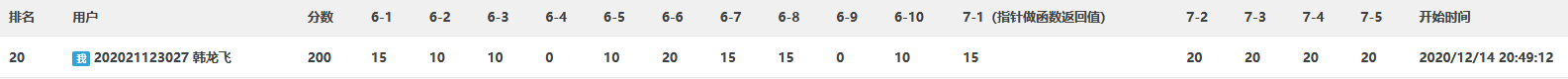## 1.1 指针定义、指针相关运算、指针做函数参数

※为何使用指针

### 1.指针的定义

• 假设变量p位于2000单元，该单元中存放变量x的地址1000，若取出变量p的值1000就可以访问内存1000单元，实现对变量x的操作，也就是通过p间接访问变量x。这种专门用来存放变量地址的变量是指针。

• 一般形式：类型名 *指针变量名

• 当指针声明符*在定义指针变量时被使用，说明被定义的变量是指针。

• 星号不是运算符，故想要对指针做处理时，前面不需要带*

• 定义多个指针变量时，每个指针变量前都必须加上*。

### 2.指针相关运算

①取地址运算和间接访问运算

int* p,a=3;
p=&a;


②赋值运算

int a=3,*p1,*p2;
p1=&a;
p2=p1;


③指针间的加减运算

④赋值

### 3.指针做函数参数

    void Swap1(int *p, int *q)
{
int buf;
buf = *p;
*p = *q;
*q = buf;
return;
}
void Swap2(int a, int b)
{
int buf;
buf = a;
a = b;
b = buf;
return;
}


## 1.2 字符指针

①字符指针：指向字符型数据的指针变量。每个字符串在内存中都占用一段连续的存储空间，并有唯一确定的首地址。即将字符串的首地址赋值给字符指针，可让字符指针指向一个字符串

char *ptr = "Hello";//将保存在常量存储区的"Hello"的首地址赋值给ptr

char *ptr;
ptr = "Hello";//是等价的，注意不能理解为将字符串赋值给ptr


char str = "Hello";
char *ptr = str;//数组名代表数组的首地址
/*等价于*/
char *ptr;
ptr = str;//等价于ptr = &str;将数组的首地址赋给字符指针ptr


④对于数组名str，不能使用str++操作使其指向字符串中的某个字符，因为数组名是一个地址常量，其值是不能被改变的。

*(ptr+i)：字符串中第i+1个字符，相当于*(str+i)，即str[i]



⑤字符串的长度（指字符串所含的字符个数，但不包括最后的’\0’）与字符数组的大小（指字符串所包含的字符个数加上’\0’，故+1）不一样。

for(i = 0;str[i] != '\0'; i++ )
{
printf("%c",str[i]);//常用借助字符串结束标志'\0'识别字符串的结束
}
scanf("%s",str);//表示读入一个字符串，直到遇到空白字符（空格、回车符、制表符）为止,如果输入带有空格的字符串,只会读到空格以前而空格以后不会读入
printf("%s",str);//表示输出一个字符串，直到遇到字符串结束标志'\0'为止（注意这里可以带有空格输出）


※常用的字符串处理函数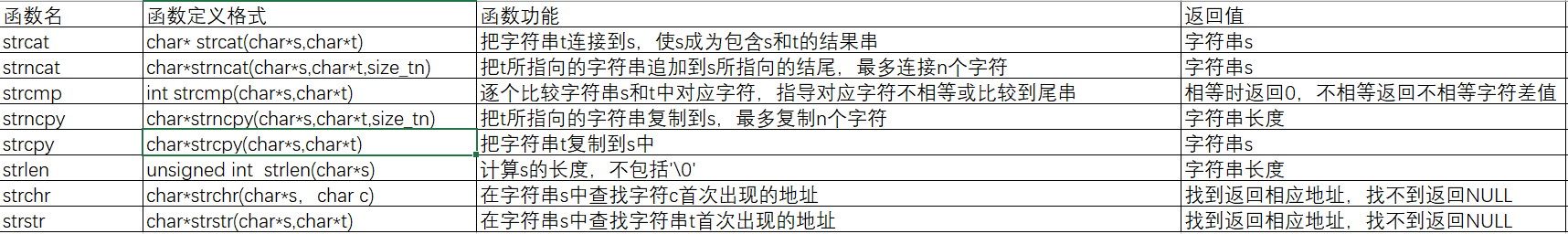## 1.4 动态内存分配

• 1.从栈区(stack)上分配
• 在执行函数调用时，系统在栈上为函数内的局部变量及形参分配内存，函数执行结束时自动释放这些内存。
• 2.从堆区(heap)上分配
• 在程序运行期间，用动态内存分配函数来申请的内存都是从堆上分配的，动态内存的生存期由程序员决定。
/*动态存储分配函数*/
void *malloc(unsigned size)//动态存储分配函数
//功能：在内存的动态存储区中分配一连续空间，其长度为size。申请成功，则返回指向所分配内存空间的起始地址指针；失败，则返回NULL。
void *calloc(unsigned n,unsigned size)//计数动态储存分配函数
//功能：在内存的动态存储区中分配n个连续空间，每一个存储空间的长度为size，分配后把存储块全部初始化为0。申请成功，则返回指向所分配内存空间的起始地址指针；失败，则返回NULL。
void free(void *ptr)//动态存储释放函数
//功能：释放由动态存储分配函数申请到的整块内存空间。
void *realloc(void *ptr.unsigned size)//分配调整函数
//功能：更改以前的存储分配。

/*动态分配n个整型大小的空间*/
if((p=(int *)malloc(n*sizeof(int)))==NULL)
{
printf("NOT able to allocate memory\n");
exix(1);
}


## 1.5 指针数组及其应用

• 1.定义

• 一般形式类型名 *数组名[数组长度];

• 指针数组中的每个元素都是指针类型，用于存放内存地址。

• 2 多个字符串用二维数组表示和用指针数组表示区别

• 二维字符数组一旦定义，那么每个字符串的最大长度、首地址都不能改变了

• 字符指针数组是存放字符指针的数组。由于它仅用来存放指针，所以它指向的每个字符串的首地址可以改变，字符串最大长度也可以改变。

• 举例：

char str={"abc","abcd","aaaa","ad","k"};
str到str五个字符串的最大长度被限为（5-1）=4，注意处理字符串时不应溢出。由于每个字符串的地址已经确定，所以str="news";是不允许的

char* str;
str="Welcome!";



## 1.7 行指针、列指针

char color = { "red","blue","yellow","green","black" };
char (*pc);
pc = color;
printf("%s\n", *(pc + 1));
printf("%c\n",*(*(pc+1)+2));


blue
u


# 2.PTA实验作业

## 2.1 删除字符串中的子串(https://pintia.cn/problem-sets/1333954783207010304/problems/1333954910390890510)

### 2.1.1 伪代码

定义字符数组s、t、q

while(p=strstr(s,t))//找到子串首字符在字符串中的地址
do while(*(p+lent)!='\0')//字符前移
do 令字符前移至遍历自子串首字符位置之后的字符串到结束符
end while
删除已前移的多余字符
end while



### 2.1.2 代码截图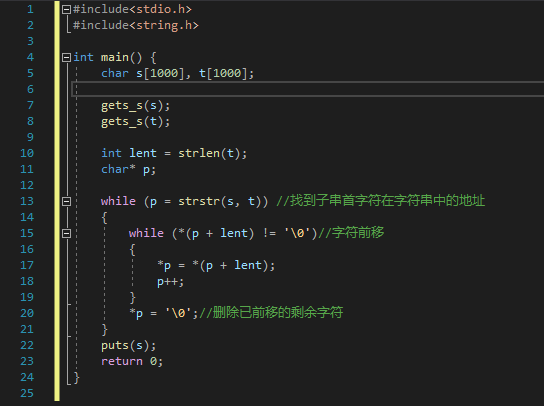### 2.1.3同学代码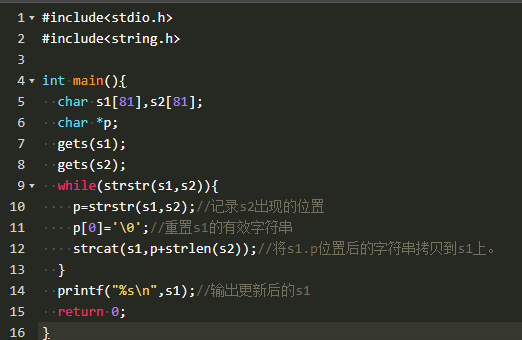## 2.2 合并2个有序数组(https://pintia.cn/problem-sets/1333954783207010304/problems/1333954910390890504)

### 2.2.1 伪代码

merge(指针a, 整型变量m, 指针b, 整型变量n)/*用于归纳并排序的函数*/

while i<m&&j<n
if *a>=*b
then a[k]=*b
b++
j++
k++
else a[k]=*p
p++
i++
k++
end if
end while
if i<m
while k<=m+n-1
do a[k]=*p
p++
k++
end while
end if
if j<n
while k<=n+m-1
then a[k]=*b
b++
k++
end while
end if
for i=1 to m+n-1
do for j=i+1 to m+n-1
do if a[i]>a[j]
then temp=a[i]
a[i]=a[j]
a[j]=temp
end if
end for
end for
printArray(指针arr, 整型变量arr_size)/*用于输出的函数*/

for i=0 to arr_size-1
if i=0
then 输出arr[i](不带空格)
else 输出 arr[i]（带空格）
end if
end for



### 2.2.2 代码截图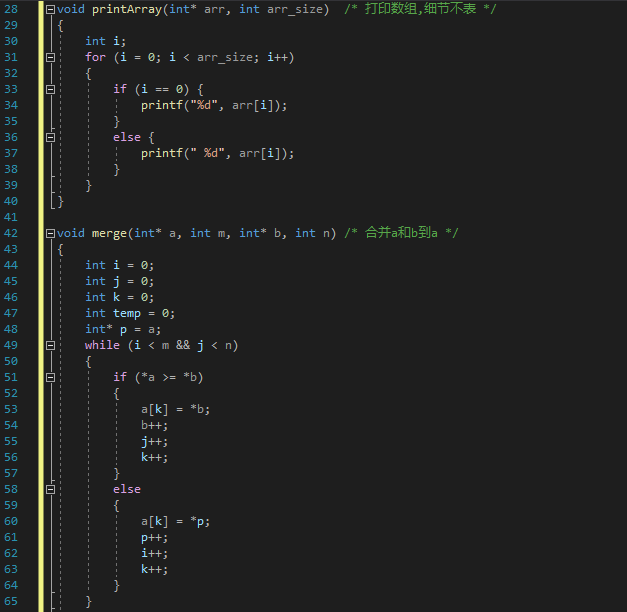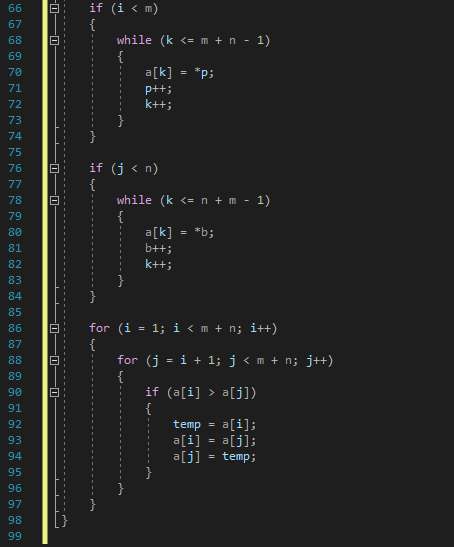### 2.2.3同学代码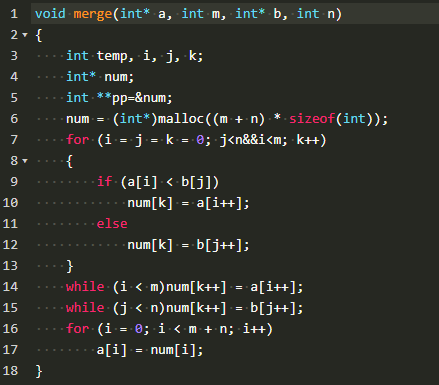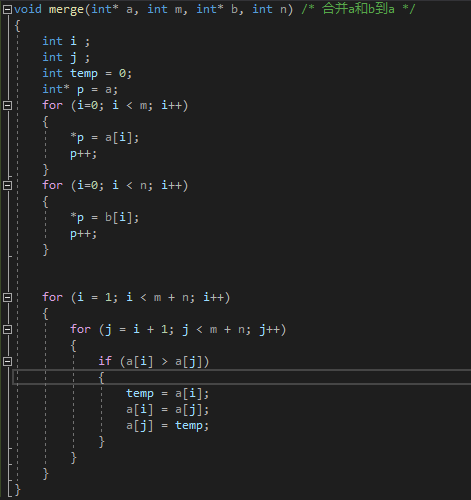## 2.3 说反话-加强版(https://pintia.cn/problem-sets/1333954783207010304/problems/1333954910390890509)

### 2.3.1 伪代码

定义字符数组a

while(*p!='\n'&&*p!='\0')
do p++
end while

while(q!=a)
do if (*q==' ')
then if (* (q + 1) != ' ' && *(q + 1) != '\0')
then if (flag==1)
then 输出q+1后的字符串
令flag=0
else 输出q后的字符串
end if
*q='\0'
end if
end if
q--
end while
if (*a!=' ')
then if (flag == 1)
then 输出q + 1后的字符串
令flag = 0
else 输出q后的字符串
end if
end if


### 2.3.2 代码截图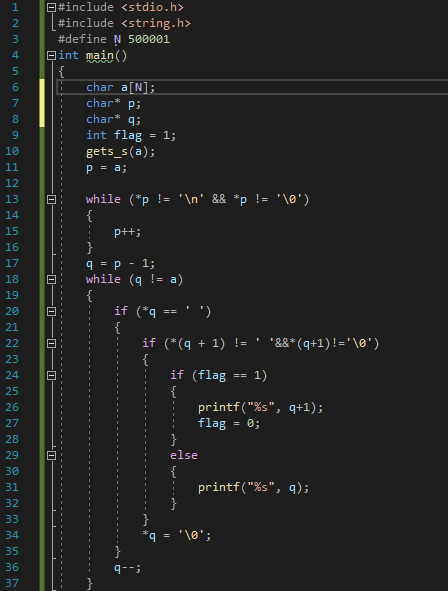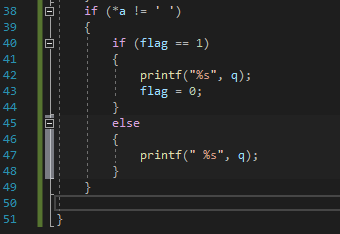### 2.3.3和超星视频做法区别

※知识点

posted @ 2020-12-27 14:57  箱推人  阅读(166)  评论(0编辑  收藏  举报